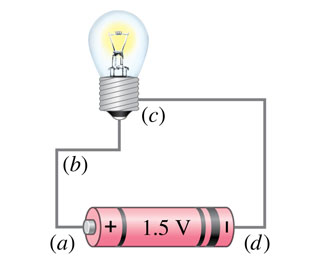# Problem: Where in the circuit of (Figure 1) is the current the largest? The current is the same at all points?

###### FREE Expert Solution

The connection is in series.

93% (289 ratings)###### Problem Details

Where in the circuit of (Figure 1) is the current the largest? The current is the same at all points?